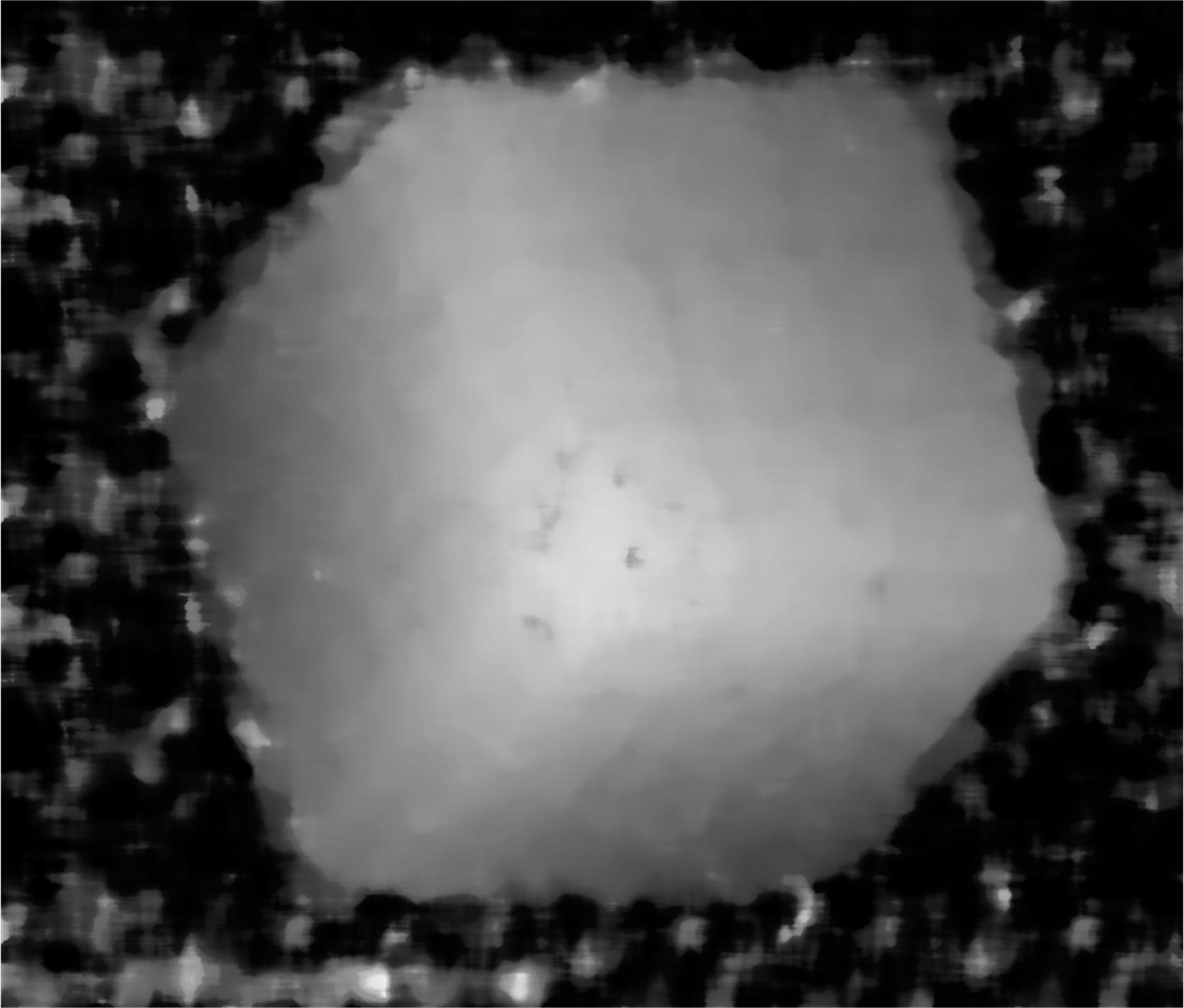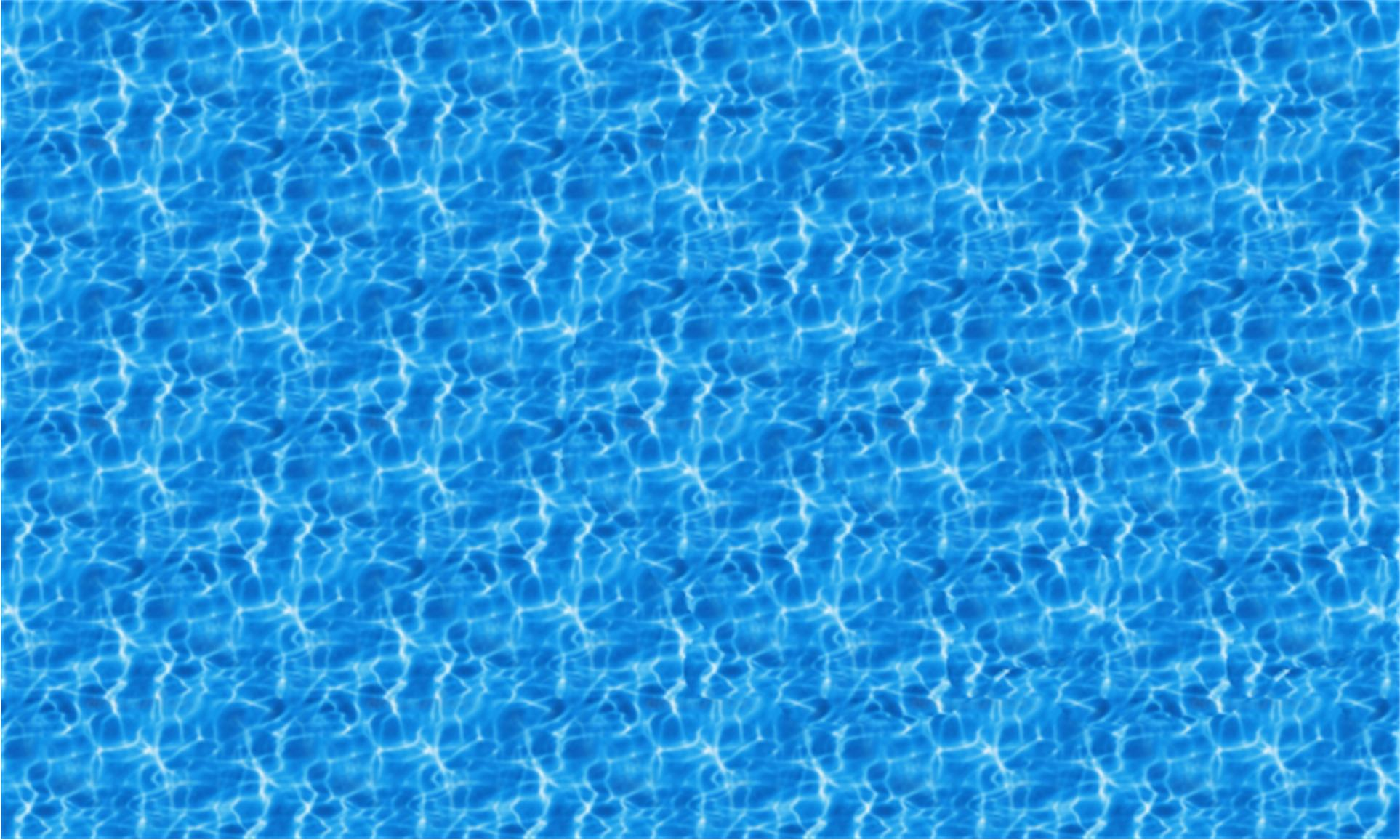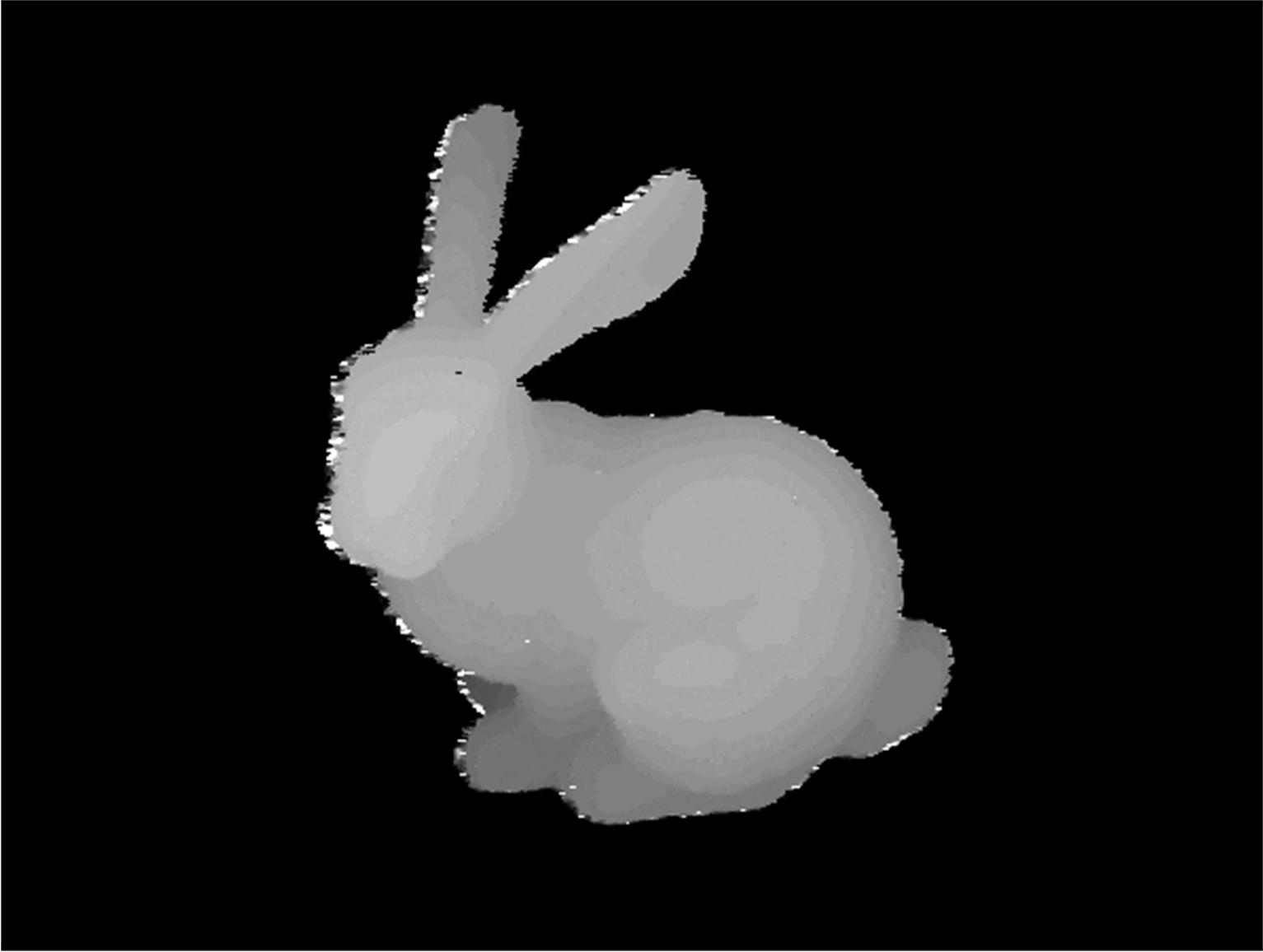# Stereogram in Mathematica: inverse question

I've seen in this wonderful post that it's possible to create a stereogram in Mathematica.

But I didn't found anything regardless the inverse problem. Given a stereogram, is possible to retrieve the hidden image (heightmap)?

For example, given this image:Is possible somehow to retrieve its height map?An ugly but working solution:First we should estimate the period of the background patter:

shift = Block[{img = ste, x, corr},
corr =
ImageData[
ImageCorrelate[ImageAssemble[{img, img}], img,
FindPeaks[-Norm /@ corr, 10][[2, 1]] - 1];


This code do not always work, so you might want to take a look at corr and choose a proper shift value manually. Then we manually set up the maximum possible height of this stereogram, symbolized by maxdist.

sft = Round[shift/2];
maxdist = 20;


Then, point by point, we compare pixel {i-sft+h,j} and {i+sft-h,j}, for fixed i and j, we choose h which minimize the difference between these two pixels. However, due to interpolation issues, we might want to interpolate the data.

subdiv[lst_, n_] :=
Prepend[Catenate[Rest[Subdivide[##, n]] & @@@ Partition[lst, 2, 1]],
lst[]]
myMedianFilter[dat_, n_] :=
MovingMedian[Join[Reverse@dat[[;; n]], dat, Reverse@dat[[-n ;; -1]]],
2 n + 1]
hdat = Block[{div = 20, kl = 2, dattemp},
dattemp = (Table[
TakeSmallest[(Norm /@
subdiv[Table[#[[i - sft - 1 + j]] - #[[i + sft + 1 -
j]], {j, 0, maxdist + 2}], div]) -> "Index",
1][], {i, sft + 2, Length[#] - sft - 2}] - 1.)/div - 1;
If[Abs[#1 - #2] < .2 #3 + .5, #1, #2] &, {dattemp,
myMedianFilter[dattemp, kl],
StandardDeviationFilter[dattemp, kl]}]
] & /@ ImageData[ste];

MedianFilter[Image[hdat/17], 10]


Here measures are taken to filter out anomolies, however, they don't always work... might require some tweak here.

With better quality images or self-generated images, the result can be much more satisfying. For example, for this image:the result will be:• That's wonderful!!! – Jepessen Mar 14 at 8:42
• @Jepessen not quite, there should be plenty of places to optimize, and the speed could definitely be improved greatly…… – Wjx Mar 16 at 8:21